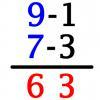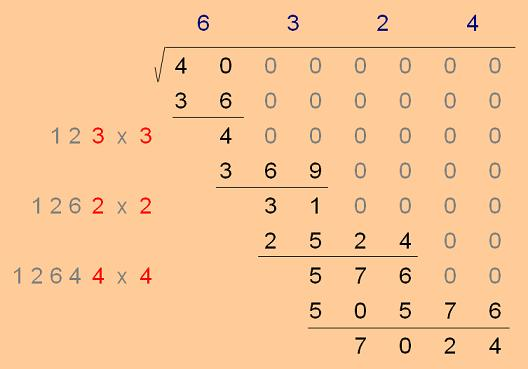#### You may also like### Triangle Incircle Iteration

Keep constructing triangles in the incircle of the previous triangle. What happens?### Vedic Sutra - All from 9 and Last from 10

Vedic Sutra is one of many ancient Indian sutras which involves a cross subtraction method. Can you give a good explanation of WHY it works?### Tournament Scheduling

Scheduling games is a little more challenging than one might desire. Here are some tournament formats that sport schedulers use.

# Unusual Long Division - Square Roots Before Calculators

##### Age 14 to 16 Challenge Level:

Well done Carol in Leeds and others. This really is right at the top end of Stage 4 material and takes some following. Most people would need to go over the argument below several times, probably taking breaks and coming back again. There are quite a lot of numbers to keep track of so stay patient with yourself and here goes :

The square roots of $40$, or $4000$, or $0.4$ all contain the same run of digits, just shifted left or right.

When a number is reduced or increased by a factor of ten the square of that number is reduced or increased by a factor of one hundred.

$4000$ reduces by a factor of one hundred to become $40$ so a factor of ten will connect their square roots.

So if I knew what the square root of $4000$ was, the square root of $40$ would be the same but with the digits all one position lower (to the right). Now to work.

This method is about deciding, one by one, what each digit is.The square root of $40$ starts with a six and then becomes decimal, so the square root of $4000$ starts with sixty-something, before becoming decimal.

And it's that 'something' digit which I need to find.

I want the whole number whose square is as close as possible to $4000$.

I know it's a two digit number and I know the first of those digits is six. If $x$ stands for the second digit then I'm trying to make $(60 + x)^2$ as close as possible to $4000$.

Written without the bracket $(60 + x)^2$ is $60^2 + 2.60.x + x^2$ so the challenge is to pick $x$ so that $2.60.x + x^2$ is as close as possible to $4000 - 3600$

$2.60.x + x^2$ is the same as $x(120 + x)$ and that matches the 'one hundred and twenty something, times something, to be close to $400$' in the second stage of working in the method.

The answer was three. $123 \times3$ is $369$

If $63$ is the whole number part of the square root of $40 00$, what about the square root of $40 00 00$ ? (the gaps in the number help me think)

The whole number part will have three digits : six, then three, then something else.

Reasoning like before, I need to get $(630 + x)^2$ as close as possible to $400000$

Writing that without the brackets is $630^2 + 2.630.x + x^2$

From which I can see that I need $1260x + x^2$ to be as close as possible to $400000 -630^2$

Or rather, I need to find the digit $x$ so that $x(1260 + x)$ is as close as possible to $3100$.

This corresponds to the third stage of working in the method. And the answer is two. $1262 \times2$ is $2524$

Now for something important : I notice that although $3100$ is the number in the working, this method for finding a square root doesn't do $400000 - 630^2$ directly to get it.The subtraction isn't $630^2$ straight off, that's $396900$, but rather an accumulation of subtractions : $36(0000)$ and $369(00)$, the zeros are needed to keep the correct column positions.
Let's look at all the subtractions shown in the working so far : $36, 369, 2524,$ and $50576$

$123 \times3$ is $3(2 \times60 + 3)$ and came to $369$
but $3(2 \times60 + 3)$ is the expanded bit of $(60 + 3)^2$ without the $60^2$

$1262 \times2$ is $2(2 \times630 + 2)$ and came to $2524$
but $2(2 \times630 + 2)$ is the expanded bit of $(630 + 2)^2$ without the $630^2$

$12644 \times4$ is $4(2 \times6320 + 4)$ and came to $50576$
but $4(2 \times6320 + 4)$ is the expanded bit of $(6320 + 4)^2$ without the $6320^2$